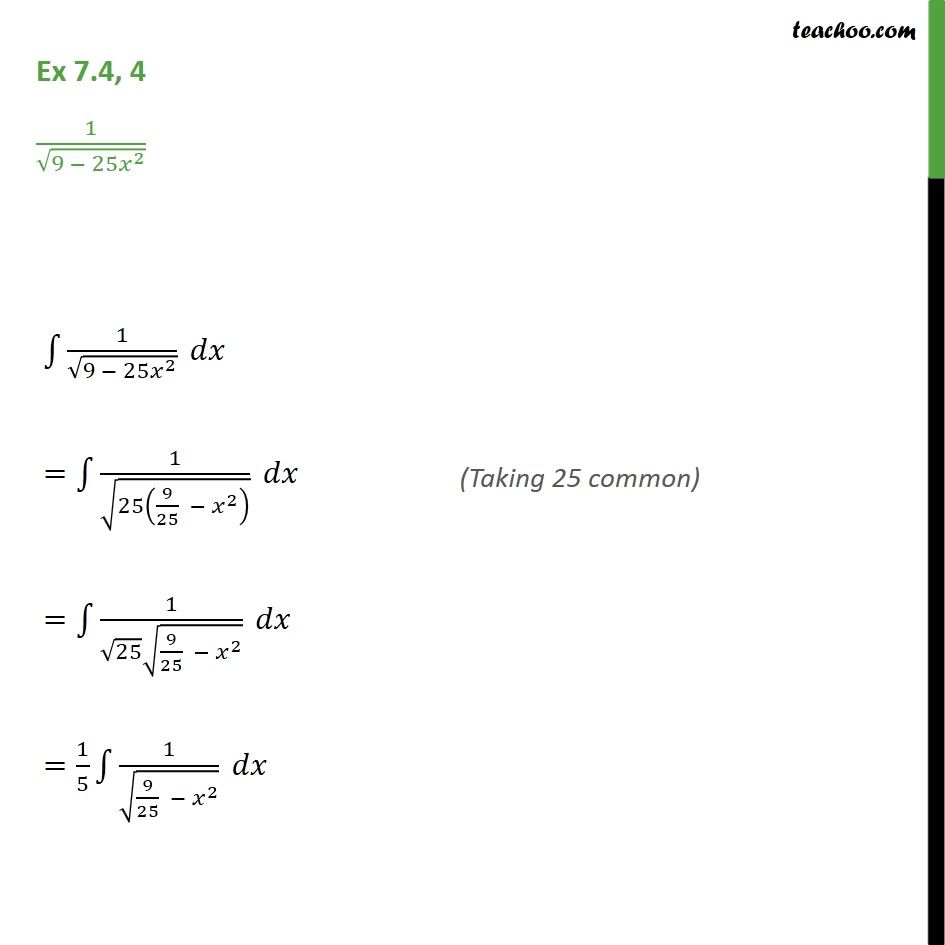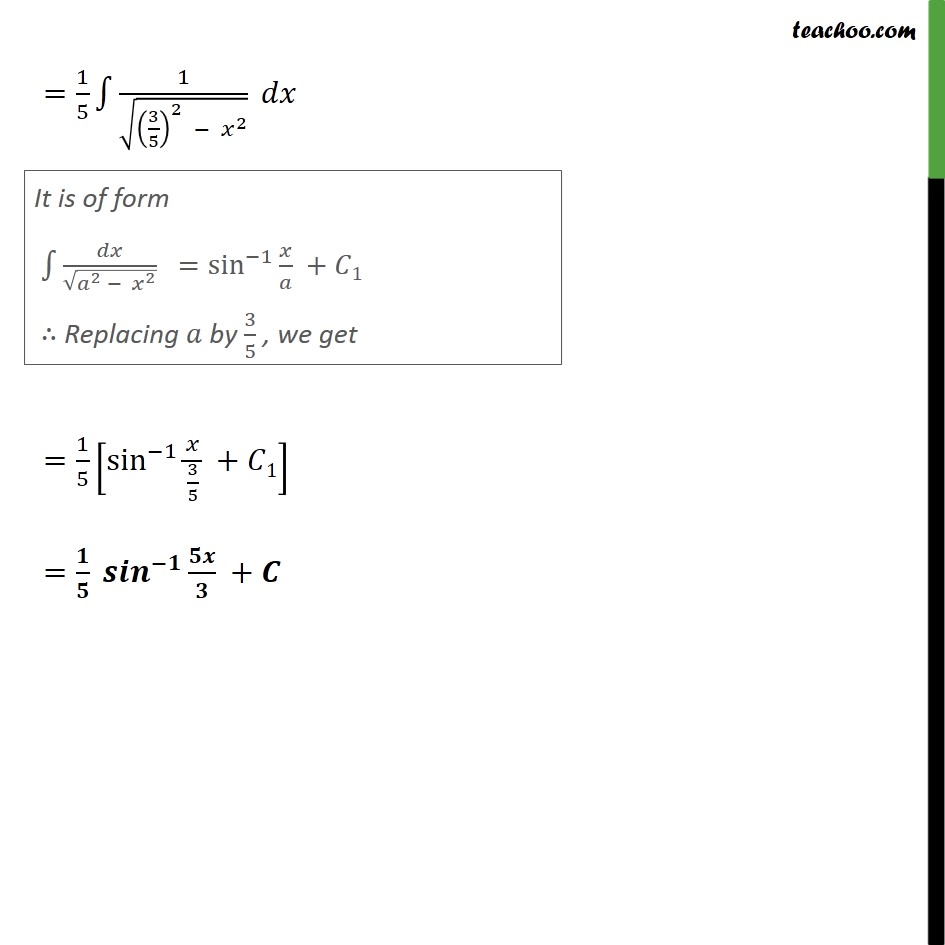1. Chapter 7 Class 12 Integrals (Term 2)
2. Concept wise
3. Integration by specific formulaes - Formula 5

Transcript

Ex 7.4, 4 1 9 25 2 1 9 25 2 = 1 25 9 25 2 = 1 25 9 25 2 = 1 5 1 9 25 2 = 1 5 1 3 5 2 2 = 1 5 sin 1 3 5 + 1 = +

Integration by specific formulaes - Formula 5